Next: 8 From C To Up: Introduction to Object-Oriented Programming Previous: 6 Even More Object-Oriented

Subsections

# 7 Introduction to C++

Peter Müller
pmueller@uu-gna.mit.edu

This section is the first part of the introduction to C++. Here we focus on C from which C++ was adopted. C++ extends the C programming language with strong typing, some features and - most importantly - object-oriented concepts.

# 7.1 The C Programming Language

Developed in the late 1970s, C gained an huge success due to the development of UNIX which was almost entirely written in this language . In contrast to other high level languages, C was written by programmers for programmers. Thus it allows sometimes, say, weird things which in other languages such as Pascal are forbidden due to its bad influence on programming style. Anyway, when used with some discipline, C is as good a language as any other.

The comment in C is enclosed in /* ... */. Comments cannot be nested.

## 7.1.1 Data Types

Table 7.1 describes the built-in data types of C. The specified Size is measured in bytes on a 386 PC running Linux 1.2.13. The provided Domain is based on the Size value. You can obtain information about the size of a data type with the sizeof operator.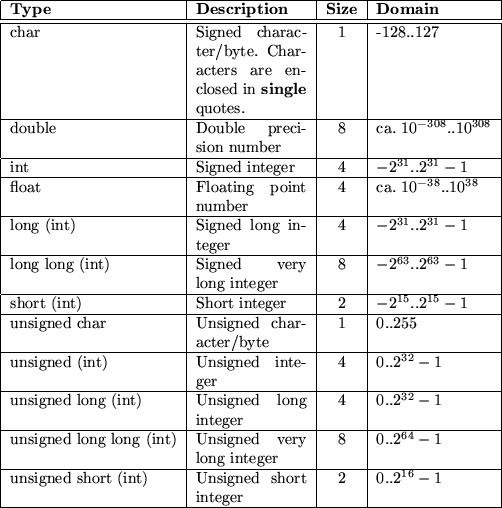Variables of these types are defined simply by preceeding the name with the type:

  int an_int;
float a_float;
long long a_very_long_integer;


With struct you can combine several different types together. In other languages this is sometimes called a record:

  struct date_s {
int day, month, year;


The above definition of aDate is also the declaration of a structure called date_s. We can define other variables of this type by referencing the structure by name:

  struct date_s anotherDate;


We do not have to name structures. If we omit the name, we just cannot reuse it. However, if we name a structure, we can just declare it without defining a variable:

  struct time_s {
int hour, minute, second;
};


We are able to use this structure as shown for anotherDate. This is very similar to a type definition known in other languages where a type is declared prior to the definition of a variable of this type.

Variables must be defined prior to their use. These definitions must occur before any statement, thus they form the topmost part within a statement block.

## 7.1.2 Statements

C defines all usual flow control statements. Statements are terminated by a semicolon ;''. We can group multiple statements into blocks by enclosing them in curly brackets. Within each block, we can define new variables:

  {
int i;      /* Define a global i */
i = 1;      /* Assign i the value 0 */
{           /* Begin new block */
int i;    /* Define a local i */
i = 2;    /* Set its value to 2 */
}           /* Close block */
/* Here i is again 1 from the outer block */
}


Table 7.2 lists all flow control statements: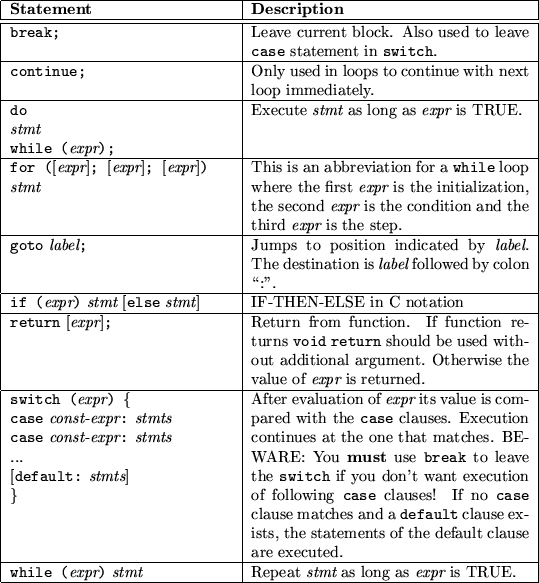The for statement is the only statement which really differs from for statements known from other languages. All other statements more or less only differ in their syntax. What follows are two blocks which are totally equal in their functionality. One uses the while loop while the other the for variant:

  {
int ix, sum;
sum = 0;
ix = 0;            /* initialization */
while (ix < 10) {  /* condition */
sum = sum + 1;
ix = ix + 1;     /* step */
}
}

{
int ix, sum;
sum = 0;
for (ix = 0; ix < 10; ix = ix + 1)
sum = sum + 1;
}


To understand this, you have to know, that an assignment is an expression.

## 7.1.3 Expressions and Operators

In C almost everything is an expression. For example, the assignment statement ='' returns the value of its righthand operand. As a side effect'' it also sets the value of the lefthand operand. Thus,

  ix = 12;


sets the value of ix to 12 (assuming that ix has an appropriate type). Now that the assignment is also an expression, we can combine several of them; for example:

  kx = jx = ix = 12;


What happens? The first assignment assigns kx the value of its righthand side. This is the value of the assignment to jx. But this is the value of the assignment to ix. The value of this latter is 12 which is returned to jx which is returned to kx. Thus we have expressed

  ix = 12;
jx = 12;
kx = 12;


in one line.

Truth in C is defined as follows. The value 0 (zero) stands for FALSE. Any other value is TRUE. For example, the standard function strcmp() takes two strings as argument and returns -1 if the first is lower than the second, 0 if they are equal and 1 if the first is greater than the second one. To compare if two strings str1 and str2 are equal you often see the following if construct:

  if (!strcmp(str1, str2)) {
/* str1 equals str2 */
}
else {
/* str1 does not equal str2 */
}


The exclamation mark indicates the boolean NOT. Thus the expression evaluates to TRUE only if strcmp() returns 0.

Expressions are combined of both terms and operators. The first could be constansts, variables or expressions. From the latter, C offers all operators known from other languages. However, it offers some operators which could be viewed as abbreviations to combinations of other operators. Table 7.3 lists available operators. The second column shows their priority where smaller numbers indicate higher priority and same numbers, same priority. The last column lists the order of evaluation.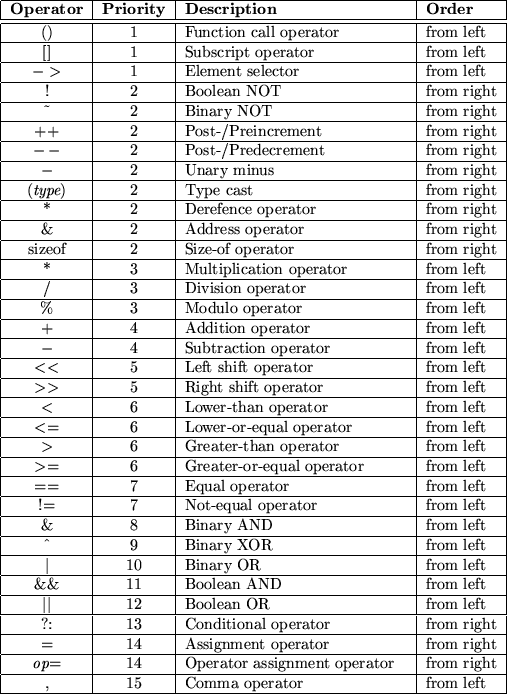Most of these operators are already known to you. However, some need some more description. First of all notice that the binary boolean operators &, ^ and | are of lower priority than the equality operators == and !=. Consequently, if you want to check for bit patterns as in

  if ((pattern & MASK) == MASK) {
...
}


you must enclose the binary operation into parenthesis.

The increment operators ++ andcan be explained by the following example. If you have the following statement sequence

  a = a + 1;
b = a;


you can use the preincrement operator

  b = ++a;


Similarly, if you have the following order of statements:

  b = a;
a = a + 1;


you can use the postincrement operator

  b = a++;


Thus, the preincrement operator first increments its associated variable and then returns the new value, whereas the postincrement operator first returns the value and then increments its variable. The same rules apply to the pre- and postdecrement operator.

Function calls, nested assignments and the increment/decrement operators cause side effects when they are applied. This may introduce compiler dependencies as the evaluation order in some situations is compiler dependent. Consider the following example which demonstrates this:

  a[i] = i++;


The question is, whether the old or new value of i is used as the subscript into the array a depends on the order the compiler uses to evaluate the assignment.

The conditional operator ?: is an abbreviation for a commonly used if statement. For example to assign max the maximum of a and b we can use the following if statement:

  if (a > b)
max = a;
else
max = b;


These types of if statements can be shorter written as

  max = (a > b) ? a : b;


The next unusual operator is the operator assignment. We are often using assignments of the following form

  expr1 = (expr1) op (expr2)


for example

  i = i * (j + 1);


In these assignments the lefthand value also appears on the right side. Using informal speech we could express this as set the value of i to the current value of i multiplied by the sum of the value of j and 1''. Using a more natural way, we would rather say Multiply i with the sum of the value of j and 1''. C allows us to abbreviate these types of assignments to

  i *= j + 1;


We can do that with almost all binary operators. Note, that the above operator assignment really implements the long form although j + 1'' is not in parenthesis.

The last unusal operator is the comma operator ,. It is best explained by an example:

  i = 0;
j = (i += 1, i += 2, i + 3);


This operator takes its arguments and evaluates them from left to right and returns the value of the rightmost expression. Thus, in the above example, the operator first evaluates i += 1'' which, as a side effect, increments the value of i. Then the next expression i += 2'' is evaluated which adds 2 to i leading to a value of 3. The third expression is evaluated and its value returned as the operator's result. Thus, j is assigned 6.

The comma operator introduces a particular pitfall when using n-dimensional arrays with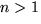. A frequent error is to use a comma separated list of indices to try to access an element:

  int matrix;  // 2-dim matrix
int i;

...
i = matrix[1,2];    // WON'T WORK!!
i = matrix;   // OK


What actually happens in the first case is, that the comma separated list is interpreted as the comma operator. Consequently, the result is 2 which leads to an assignment of the address to the third five elements of the matrix!

Some of you might wonder, what C does with values which are not used. For example in the assignment statements we have seen before,

  ix = 12;
jx = 12;
kx = 12;


we have three lines which each return 12. The answer is, that C ignores values which are not used. This leads to some strange things. For example, you could write something like this:

  ix = 1;
4711;
jx = 2;


But let's forget about these strange things. Let's come back to something more useful. Let's talk about functions.

## 7.1.4 Functions

As C is a procedural language it allows the definition of functions. Procedures are simulated'' by functions returning no value''. This value is a special type called void.

Functions are declared similar to variables, but they enclose their arguments in parenthesis (even if there are no arguments, the parenthesis must be specified):

  int sum(int to);  /* Declaration of sum with one argument */
int bar();        /* Declaration of bar with no arguments */
void foo(int ix, int jx);
/* Declaration of foo with two arguments */


To actually define a function, just add its body:

  int sum(int to) {
int ix, ret;
ret = 0;
for (ix = 0; ix < to; ix = ix + 1)
ret = ret + ix;
return ret;      /* return function's value */
} /* sum */


C only allows you to pass function arguments by value. Consequently you cannot change the value of one argument in the function. If you must pass an argument by reference you must program it on your own. You therefore use pointers.

## 7.1.5 Pointers and Arrays

One of the most common problems in programming in C (and sometimes C++) is the understanding of pointers and arrays. In C (C++) both are highly related with some small but essential differences. You declare a pointer by putting an asterisk between the data type and the name of the variable or function:

  char *strp;      /* strp is pointer to char' */


You access the content of a pointer by dereferencing it using again the asterisk:

  *strp = 'a';            /* A single character */


As in other languages, you must provide some space for the value to which the pointer points. A pointer to characters can be used to point to a sequence of characters: the string. Strings in C are terminated by a special character NUL (0 or as char '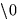'). Thus, you can have strings of any length. Strings are enclosed in double quotes:

  strp = "hello";


In this case, the compiler automatically adds the terminating NUL character. Now, strp points to a sequence of 6 characters. The first character is h', the second e' and so forth. We can access these characters by an index in strp:

  strp     /* h */
strp     /* e */
strp     /* l */
strp     /* l */
strp     /* o */
strp     /* \0 */


The first character also equals *strp'' which can be written as *(strp + 0)''. This leads to something called pointer arithmetic and which is one of the powerful features of C. Thus, we have the following equations:

  *strp == *(strp + 0) == strp
*(strp + 1) == strp
*(strp + 2) == strp
...


Note that these equations are true for any data type. The addition is not oriented to bytes, it is oriented to the size of the corresponding pointer type!

The strp pointer can be set to other locations. Its destination may vary. In contrast to that, arrays are fix pointers. They point to a predefined area of memory which is specified in brackets:

  char str;


You can view str to be a constant pointer pointing to an area of 6 characters. We are not allowed to use it like this:

  str = "hallo";   /* ERROR */


because this would mean, to change the pointer to point to 'h'. We must copy the string into the provided memory area. We therefore use a function called strcpy() which is part of the standard C library.

  strcpy(str, "hallo"); /* Ok */


Note however, that we can use str in any case where a pointer to a character is expected, because it is a (fixed) pointer.

## 7.1.6 A First Program

Here we introduce the first program which is so often used: a program which prints Hello, world!'' to your screen:

  #include <stdio.h>

/* Global variables should be here */

/* Function definitions should be here */

int
main() {
puts("Hello, world!");
return 0;
} /* main */


The first line looks something strange. Its explanation requires some information about how C (and C++) programs are handled by the compiler. The compilation step is roughly divided into two steps. The first step is called preprocessing'' and is used to prepare raw C code. In this case this step takes the first line as an argument to include a file called stdio.h into the source. The angle brackets just indicate, that the file is to be searched in the standard search path configured for your compiler. The file itself provides some declarations and definitions for standard input/output. For example, it declares a function called put(). The preprocessing step also deletes the comments.

In the second step the generated raw C code is compiled to an executable. Each executable must define a function called main(). It is this function which is called once the program is started. This function returns an integer which is returned as the program's exit status.

Function main() can take arguments which represent the command line parameters. We just introduce them here but do not explain them any further:

  #include <stdio.h>

int
main(int argc, char *argv[]) {
int ix;
for (ix = 0; ix < argc; ix++)
printf("My %d. argument is %s\n", ix, argv[ix]);
return 0;
} /* main */


The first argument argc just returns the number of arguments given on the command line. The second argument argv is an array of strings. (Recall that strings are represented by pointers to characters. Thus, argv is an array of pointers to characters.)

# 7.2 What Next?

This section is far from complete. We only want to give you an expression of what C is. We also want to introduce some basic concepts which we will use in the following section. Some concepts of C are improved in C++. For example, C++ introduces the concept of references which allow something similar to call by reference in function calls.

We suggest that you take your local compiler and start writing a few programs (if you are not already familiar with C, of course). One problem for beginners often is that existing library functions are unknown. If you have a UNIX system try to use the man command to get some descriptions. Especially you might want to try:

  man gets
man printf
man puts
man scanf
man strcpy
`

We also suggest, that you get yourself a good book about C (or to find one of the on-line tutorials). We try to explain everything we introduce in the next sections. However, there is nothign wrong with having some reference at hand.Next: 8 From C To Up: Introduction to Object-Oriented Programming Previous: 6 Even More Object-Oriented
P. Mueller
8/31/1997